##Eleven Point Conic

Given four points {A, B, C, D} in general position and a line (d) also in general position relative to the points (not passing through anyone of them) there is a conic (c) containing eleven remarkable points, defined through the given data. These are:
- The three diagonal points P, Q, R of ABCD.
- The six conjugates U1', ... , U6' of the intersection points U1,..., U6 of (d) with the sides of ABCD.
- The two (if existent) fixed points X1, X2 of the [Desargues involution] on the line (d), defined through the conics family generated by the pairs of lines: (AB, CD) and (BC, DA).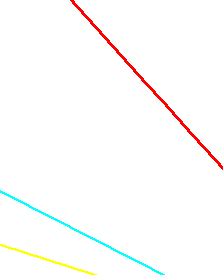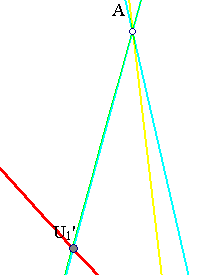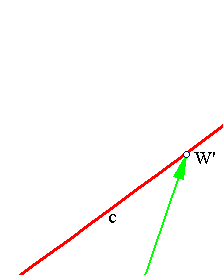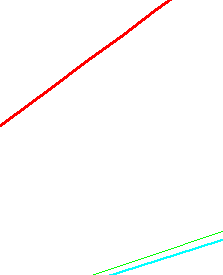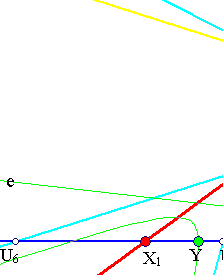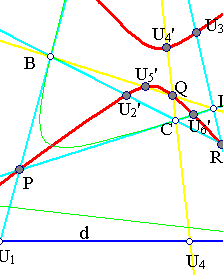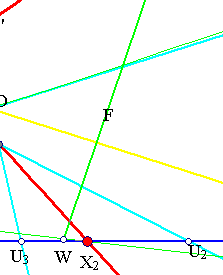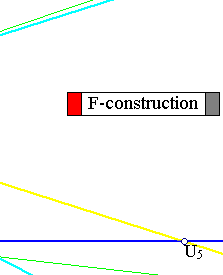c is the image of the line (d) through the quadratic transformation F, defined by the bundle of conics generated by the two pairs of intersecting lines (AB,CD) and (BC,DA). As usual for this transformation, the images of lines are conics passing through the singular points of the bundle, which are P, Q, R. To see that U1',...,U6' should be on that conic, consider the definition of the corresponding quadratic transformation. By it, the image of a point like, say P=U3, is the common point of all polars of P w.r. to all conics of the family. But since all these conics pass through {A,B,C,D} the intersection point of all the polars of P must coincide with U3', which is harmonic conjugate to U3 w.r. to A,D.
That c should pass through X1, X2 follows from the fact that F interchanges them. To see this consider a typical member of the family c'(Y) passing through the point Y of line d. Then construct the polar (e(Y)) of point X1 w.r. to c'(Y). When Y = X1, the polar is identical with line d. When Y = X2, the polar of X1 w.r. to c'(Y) passes through the contact points of c' with the tangents from X1, one of them being d, thus the polar of X1 w.r. to c'(X2) passes also from X2.
Note that {A,B,C,D} are the fixed points of the quadratic transformation F.
Note also that poins X1, X2 were encountered also in the problem of determining the conics passing through four points {A,B,C,D} and tangent to a given line (d), discussed in FourPtsAndTangent.html .

### See Also

DesarquesInvolution.html
FourPtsAndTangent.html
Quadratic_Transformation.html
Quadratic_Transformation2.html

### References

Berger, M Geometry I, II Paris, Springer Verlag, 1987, vol. II, par. 16.5.5.1 p. 198.

 Produced with EucliDraw© http://users.math.uoc.gr/~pamfilos/